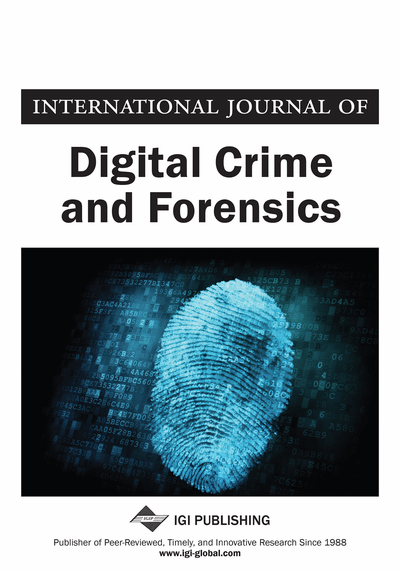# Secure Robust Hash Functions and Their Applications in Non-interactive Communications

Qiming Li (Institute for Infocomm Research, Singapore) and Sujoy Roy (Institute for Infocomm Research, Singapore)
DOI: 10.4018/jdcf.2010100104
Available
\$37.50
No Current Special Offers

## Abstract

A robust hash function allows different parties to extract a consistent key from a common fuzzy source, e.g., an image gone through noisy channels, which can then be used to establish a cryptographic session key among the parties without the need for interactions. These functions are useful in various communication scenarios, where the security notions are different. The authors study these different security notions in this paper and focus on forgery attacks, where the objective of the attack is to compute the extracted key (hash value) of a given message. This paper will examine information-theoretical security against forgery under chosen message attacks. The authors prove that it is not possible due to the entropy of the hash value of a given message can be reduced arbitrarily when sufficient message/hash pairs have been observed. In this regard, the authors give a computationally secure scheme, where it is computationally infeasible to compute the hash value even when its entropy may not be high.
Article Preview
Top

Swaminathan et al. (2006) give a security model for analyzing the security of a robust hash, where they use differential entropy as a measure of security for randomized image features. It is also suggested that the security of a hashing scheme should be measured by the conditional entropy of the hashing key, when the hashing algorithm, and pairs of images and their hashes are known. We formalize this notion and show that such information-theoretic security is impossible.

The robust hash proposed by Swaminathan et al. (2006) is specifically designed for an authentication application. There are existing works that analyze security models for authentication application scenarios. Some examples of proposed scenarios are as follows. Ge et al. (2006) analyze a scenario where sends a message and an approximate message authentication code (AMAC) to for verification. The AMAC serves as a keyed similarity-preserving function. Another scenario as discussed by Li and Chang (2006) is when sends a message, some helper data and a cryptographic MAC to, where the noise during communication can be corrected using the helper data. In both cases, and share a secret key. If public-private key pairs are used instead of secret keys in these scenarios, it would become a digital signature. Another scenario focusing on key extraction is studied by Dodis et al. (2004) where and have access to content and its corrupted version respectively. extracts a key and some helper data which is sent to . uses and the helper data to generate another key , and if and are close enough. In all the above three scenarios there is need for communication between and. In the scenario proposed in this paper there is no communication between and.

We highlight that the scenario considered in this work represents a more general applicability of robust hash. In fact Mihcak and Venkatesan (2001) also propose a robust hashing algorithm to extract a consistent key from certain content but for watermarking application. Note that we also extract a consistent key, which can be used for authentication. Hence it is interesting to study the security of robust hash under the proposed scenario.

Top

## Definitions And Notations

Let be the message space. We do not impose any constraint on. For example, it can be the set of feature vectors from a database of images. The distribution of the message can be either discrete or continuous. Here we mainly use discrete distributions and entropies as examples. Similar definitions and proofs based on differential entropies can be easily adapted. Let be a distance function defined over. We consider the following definitions of hash functions and their properties.

• Definition 1 (Hash Function) A hash function is an efficiently computable function, , where and is some positive polynomial.

Here being “efficiently computable” means that can be computed with a polynomial time deterministic algorithm. Such a hash function may have the following properties.

• Definition 2 (Robustness) A hash function is -robust w.r.t. key length if for any such that, with probability at least.

• Definition 3 (Sensitivity) A hash function is -sensitive w.r.t. key length if for random and random such that, we have .

## Complete Article List

Search this Journal:
Reset
Volume 13: 6 Issues (2021): 1 Released, 5 Forthcoming
Volume 12: 4 Issues (2020)
Volume 11: 4 Issues (2019)
Volume 10: 4 Issues (2018)
Volume 9: 4 Issues (2017)
Volume 8: 4 Issues (2016)
Volume 7: 4 Issues (2015)
Volume 6: 4 Issues (2014)
Volume 5: 4 Issues (2013)
Volume 4: 4 Issues (2012)
Volume 3: 4 Issues (2011)
Volume 2: 4 Issues (2010)
Volume 1: 4 Issues (2009)
View Complete Journal Contents Listing